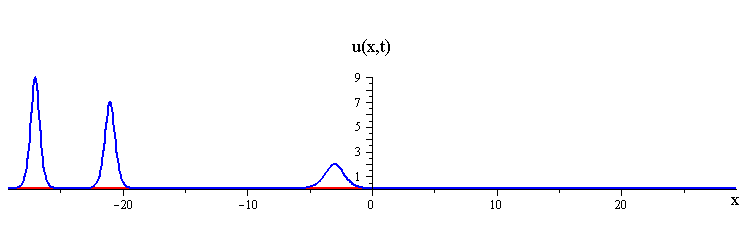# KdV Solitons

## KdV Equation ut + αuux + βuxxx = 0

The coefficients α, β in the general form of the KdV equation can be freely changed through scaling/reflection transformations on the variables u, x, t. A conventional choice is α = 6, β = 1, which eliminates awkward numerical factors in the expressions for soliton solutions.

### 1-Soliton Solution

The KdV 1-soliton solution is
u(x,t)= (c/2)/cosh2(√c ξ/2)

where c > 0 is the wave speed and ξ = xct is a moving coordinate.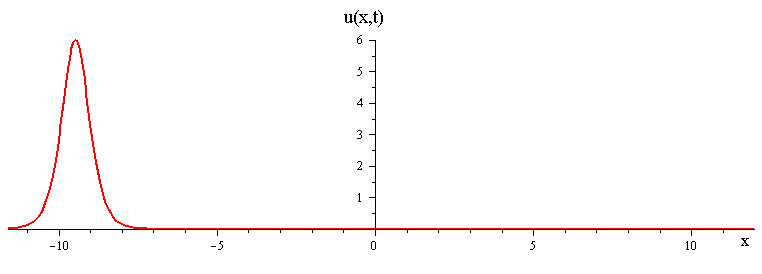The position of the wave peak at time t is x = ct.

### 2-Soliton Solution

The KdV 2-soliton solution is
u(x,t)= 2(c1c2) ( c1 cosh2(√c2 ξ2/2) + c2 sinh2(√c1 ξ1/2) )/( (√c1 − √c2) cosh((√c1 ξ1 + √c2 ξ2)/2)
+ (√c1 + √c2) cosh((√c1 ξ1 − √c2 ξ2)/2) )2

where c1 > c2 > 0 are the wave speeds and ξ1 = xc1t, ξ2 = xc2t are moving coordinates.

As t → ±, the 2-soliton solution has the asymptotic form of a linear superposition of travelling waves (i.e. single solitons) with speeds c1 and c2. The two waves wave collide such that the moment of greatest nonlinear interaction occurs at t = 0 when the profile is symmetric is symmetric around x = 0. The shape of this profile u(x,0)= u(−x,0) is characterized by its convexity u(x,0)xx|x=0 which is positive or negative depending on whether the speed ratio is greater or less than a critical value c1 / c2 = 3 (for which the convexity vanishes).

For speed ratios c1 / c2 > 3, the profile has a single peak at x = 0 and an exponentially diminishing tail as x → ±. In this case the waves interact nonlinearly by first merging at x = t = 0 and then splitting apart.
KdV merge-split interactionratio of fast to slow wave speeds is > 3

For speed ratios c1 / c2 < 3, the profile has a double peak around x = 0 and an exponentially diminishing tail as x → ±. In this case the waves interact nonlinearly by first bouncing and then exchanging shapes and speeds at x = t = 0.
KdV bounce-exchange interactionratio of fast to slow wave speeds is < 3

### Overlay of 1-soliton solutions and corresponding 2-soliton solution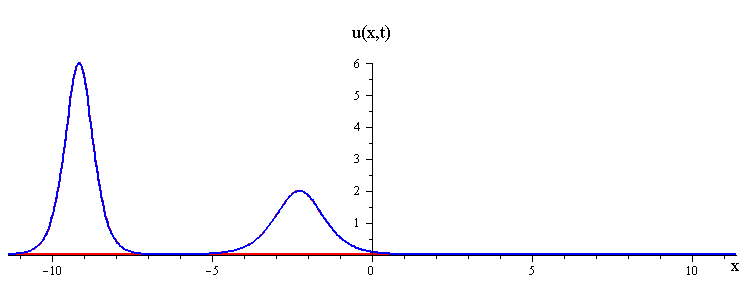ratio of fast to slow wave speeds is > 3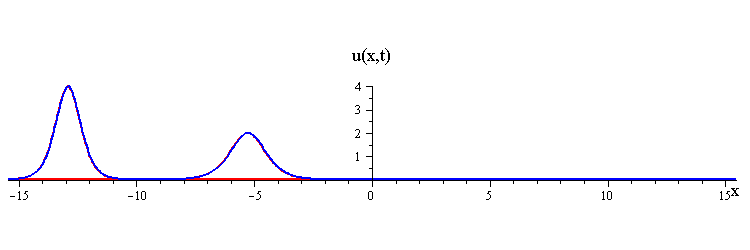ratio of fast to slow wave speeds is < 3
In both types of interactions, the waves emerging from the collision retain the shape and speed of the initial two waves, but the collision causes a position shift such that the faster wave gets shifted forward by
Δx1 = (2/k1) ln ( (k1 + k2)/(k1k2) ) > 0

and the slower wave gets shifted backward by
Δx2 = (2/k2) ln ( (k1k2)/(k1 + k2) ) < 0

where k1 = √c1 > k2 = √c2 > 0.

### Multi-soliton Collisions

Multi-soliton solutions have the same interaction features displayed by the 2-soliton solution.

### 3-Soliton Simultaneous Collision Solution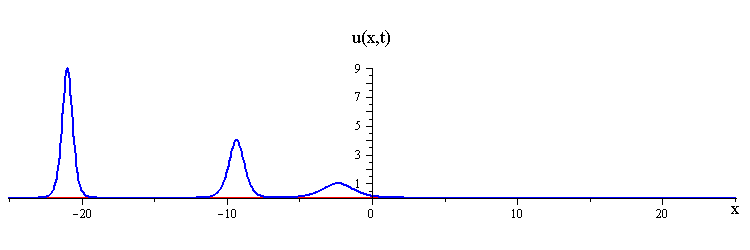All three waves collide at x = 0, t = 0.

### 3-Soliton Pair-wise Collision Solution